# Richardson extrapolation

A method for accelerating the convergence of solutions of difference problems (see Approximation of a differential boundary value problem by difference boundary value problems). The principal idea of the method consists in regarding the solutionof a convergent difference problem for fixedas a function of the vanishing parameterof the difference grid, choosing the appropriate interpolation function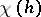based on several values of the solutionfor different, and calculating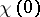which is an approximate value of the sought solution— the limit of the sequence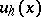as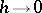. Usually, the functionis sought in the form of an interpolation polynomial in.

The method is called after L. Richardson  who was the first to apply it to improve the precision of the solution of difference problems and called it a deferred approach to the limit.

The theoretical basis of the applicability of this method is the existence of an expansion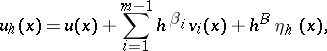where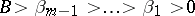, the functions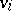are independent of, and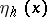are the values of a grid function which is bounded when. There are several theoretical methods for finding out whether such expansions exist or not .

Usually, linear extrapolation is used: Byvalues ofat the same pointfor different parameters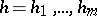one calculates the extrapolated valueby the formula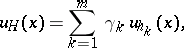where the weights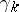are defined by the following system of equations: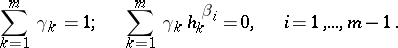If among thethere are no values which are too close to each other, then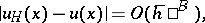where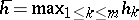, i.e. the order of convergence of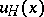to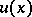asis, which is greater than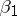— the order of convergence ofto. In two special cases there exist algorithms for calculatingwithout having to determine the coefficients:

a) in the case when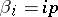,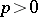,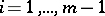, the method reduces to the interpolation of a polynomial in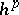, and from the Lagrange interpolation formula it follows that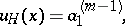where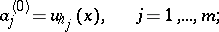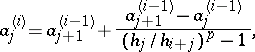(*)b) in the case when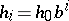,,, formula (*) is replaced byThis algorithm, called Romberg's rule (W. Romberg, 1955), is widely applied in the construction of quadrature formulas (see ). In order that for differentthe grids(see Approximation of a differential operator by difference operators) have as many nodes as possible to carry out the Richardson extrapolation, the parameters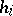are chosen as part of one of the following sequences: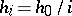,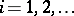;,;.

Linear extrapolation is not the only possible way. For instance, in case,, as the interpolation functionone can take rational functions of the form, where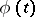,are polynomials inof degreesand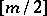, respectively. Then the result of the rational extrapolation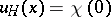can be calculated by the following recurrent procedure: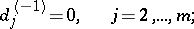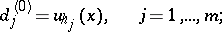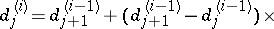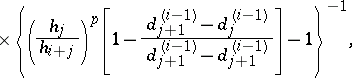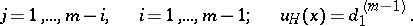Richardson extrapolation is conveniently realized on a computer, since in order to achieve high accuracy it uses the repeated solution of simple difference problems (sometimes with little modifications) of low order of approximation for which standard methods of solution and computer programs are usually well developed.